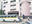Trending ▼   ResFinder# ICSE Pre-Prelims 2018 : Mathematics (Arya Vidya Mandir (AVM), Mumbai)

7 pages, 47 questions, 46 questions with responses, 205 total responses,11Aaryamonvikram Singh Vasudev C. Wadhwa Arya Vidya Mandir (VCW AVM), Mumbai 1st to 10th
+Fave Message
 Home > zenfex >   F Also featured on: School Page icseFormatting page ...

A R Y A V D Y A M A N D IR G R O U P O F S C H O o L S S td X M a th D a te 1 1 1 2 2 0 1 7 PRE . . A - P R E L IM N A R Y R E V IE W C S M ATH EM AT 2017 - M a rk s T Im e 2 8 0 % h rs . n s w e rs to th is p a p e r m u st b e ritteri o n th e p a p e r p r o v id e d s e a r a te l p y a llo w e d to w rite d u rin g th e firs t 1 5 m in u te s T h is tim e is to b e s p e n t in re a d in g th e Q u e s tio n p a e r p T h e tim e g iv e n a t th e h e a d o f th is a e r is th e tim e a llo w e d fo r w ri p p tin . Yo u w ill n o t b e . A tte A ll w m pt a q u e s tio n s fro m includng o rk in g i D u gh S e c t io n a n d a n y fo u r fr o m A The g th e a n s w e rs S e c t io n B . . w o rlr m u s t b e c le a rly s h o w n a n d m u s t b e d o n e o n th e s a m e s h e e t a s th e re s t o f th e a n s w e r O . . m is s io n o f e s s e n tia l w o rk in i l re s u l! in th e lo s s o f m a rk s g w . in te n d e d m a rk s fo r u e s tio n s o r a ris o f u e s tio n s a re q p q g iv e n in b r a c k e ts [ ] 7 h s p a p e r c o n s is ts o f s e v e n p rin te d p a g e s , . S e c t io (A tte m Q a . u e s t io n F in d In atA - (4 0 l q u e s tio n s fr o pt a M a m rk s ) t h is s e c tio n ) 1 th e v a ue o f x a nd y L ] b n R . th e fig u re . L T A D B D is , L , 3 [1 A B C D is = 6EJ a c y c lic q u a d rila te ra l a n d P T th e d ia m e te r o f th e c irc le PA B a n d z C B D . L A B D - 3 0 a n is a ta n g e n t to th e c irc le d L C D B - ' 6 0 ' . D e te rm in e . . . . 2 Scanned by CamScannerFormatting page ...

Top Contributors
to this ResPaperArya_13(34)Sunil Samuel(20)Pranav Ajinkya(16)Sparsh Agarwal(16)Formatting page ...Formatting page ...Formatting page ...Formatting page ...Formatting page ...